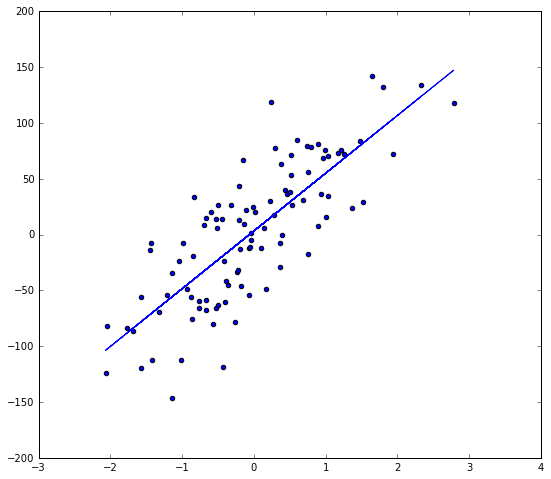# gradient descent (경사 하강법)¶

• Gradient descent 설명
• Univariate Linear Regression 에서 gradient descent (경사 하강법) 설명
• Hypothesis Function $$h_{\theta_0, \theta_1}(x) = \theta_0 + \theta_1 \cdot x$$
• Cost Function $J(\theta_0, \theta_1)$
\begin{eqnarray} J(\theta_0, \theta_1) &=& \dfrac{1}{2m} \sum_{i=1}^m \big( h_{\theta_0, \theta_1}(x^i) - y^i \big)^2 \\ &=& \dfrac{1}{2m}\sum_{i=1}^m \big( \theta_0 + \theta_1\cdot x^i - y^i \big)^2 \end{eqnarray}
• We need derivatives for both $\theta_0$ and $\theta_1$:
\begin{eqnarray} \frac{\partial}{\partial \theta_0}J(\theta_0, \theta_1) &=& \frac{1}{m} \sum_{i=1}^m (\theta_0 + \theta_1 x^{i}-y^{i}) \\ \frac{\partial}{\partial \theta_1}J(\theta_0, \theta_1) &=& \frac{1}{m} \sum_{i=1}^m (\theta_0 + \theta_1 x^{i}-y^{i})\cdot x^{i} \end{eqnarray}

#### Batch Gradient Descent¶

• 1) Pick an initial value for $\hat \theta$.
• 2) Compute $$\frac{\partial J}{\partial \theta} = \big( \frac{\partial J(\theta_0, \theta_1)}{\partial \theta_0}, \frac{\partial J(\theta_0, \theta_1)}{\partial \theta_1} \big)$$
• 3) Compute with a proper learning rate $\alpha$ $$temp_0 := \theta_0 -\alpha \frac{\partial}{\partial \theta_0}J(\theta_0, \theta_1)\\ temp_1 := \theta_1 -\alpha \frac{\partial}{\partial \theta_1}J(\theta_0, \theta_1)$$ $$\theta_0 := temp_0\\ \theta_1 := temp_1.$$

• 4) Repeat 2) and 3) until update is small or reaches iteration maximum.

#### Stochastic (or Incremental) Gradient Descent¶

• 1) Pick an initial value for $\hat \theta$.
• 2) Compute $$\frac{\partial J}{\partial \theta} = \big( \frac{\partial J(\theta_0, \theta_1)}{\partial \theta_0}, \frac{\partial J(\theta_0, \theta_1)}{\partial \theta_1} \big)$$
• 3) Compute with a proper learning rate $\alpha$ $$\theta_0 := \theta_0 -\alpha \frac{\partial}{\partial \theta_0}J(\theta_0, \theta_1)\\ \theta_1 := \theta_1 -\alpha \frac{\partial}{\partial \theta_1}J(\theta_0, \theta_1).$$

• 4) Repeat 2) and 3) until update is small or reaches iteration maximum.

• This method converges to the minimum more rapidly, but has the potential of overshooting the minimum and then oscillating around it.

#### Learning Rate control¶

• By slowly letting the learning rate $\alpha$ decrease to zero as the algorithm runs, it is also possible to ensure that the parameters will converge to the global minimum rather then merely oscillate around the minimum.

#### Local Optimum vs. Global optimum¶

• For linear regression, the cost function $J(\theta)$ does not have a local optimum other than the global optimum.• However, we need to be susceptible to local optima in general cases.#### Barch Gradient Descent - Python Code.¶

• We get $\theta_0$ and $\theta_1$ as its output:
In :
import numpy as np
import random
import sklearn
from sklearn.datasets.samples_generator import make_regression
import pylab
from scipy import stats

def gradient_descent(alpha, x, y, ep=0.0001, max_iter=10000):
converged = False
iter = 0
m = x.shape # number of samples

# initial theta
t0 = np.random.random(x.shape)
t1 = np.random.random(x.shape)

# total error, J(theta)
J = sum([(t0 + t1*x[i] - y[i])**2 for i in range(m)])

# Iterate Loop
while not converged:
# for each training sample, compute the gradient (d/d_theta j(theta))
grad0 = 1.0/m * sum([(t0 + t1*x[i] - y[i]) for i in range(m)])
grad1 = 1.0/m * sum([(t0 + t1*x[i] - y[i])*x[i] for i in range(m)])

# update the theta_temp
temp0 = t0 - alpha * grad0
temp1 = t1 - alpha * grad1

# update theta
t0 = temp0
t1 = temp1

# mean squared error
e = sum( [ (t0 + t1*x[i] - y[i])**2 for i in range(m)] )

if abs(J-e) <= ep:
print 'Converged, iterations: ', iter, '!!!'
converged = True

J = e   # update error
iter += 1  # update iter

if iter == max_iter:
print 'Max interactions exceeded!'
converged = True

return t0,t1

• Data Preparation
In :
from sklearn.datasets.samples_generator import make_regression
x, y = make_regression(n_samples=100, n_features=1, n_informative=1, noise=35)
print 'x.shape = %s, y.shape = %s' % (x.shape, y.shape)
print x[0:5]
print y[0:5]

x.shape = (100, 1), y.shape = (100,)
[[-2.04952781]
[-0.43153213]
[ 0.09858666]
[-1.01328069]
[ 1.93555916]]
[ -82.10317641 -118.249632    -11.85958046 -111.97082092   72.50576718]

• Do gradient descent!
In :
alpha = 0.01 # learning rate
ep = 0.01 # convergence criteria

# call gredient decent, and get intercept(=theta0) and slope(=theta1)
theta0, theta1 = gradient_descent(alpha, x, y, ep, max_iter=10000)
print ('theta0 = %s, theta1 = %s') %(theta0, theta1)

# check with scipy linear regression
slope, intercept, r_value, p_value, slope_std_error = stats.linregress(x[:,0], y)
print ('intercept = %s, slope = %s') %(intercept, slope)

Converged, iterations:  712 !!!
theta0 = [ 3.29752334], theta1 = [ 51.72813087]
intercept = 3.31458577872, slope = 51.8034956124

In :
import matplotlib.pyplot as plt
%matplotlib inline
fig = plt.figure(figsize=(9, 8))

[<matplotlib.lines.Line2D at 0x10dcc1ed0>]